Journal DetailsFormat
Journal
eISSN
2300-0929
First Published
19 Oct 2012
Publication timeframe
4 times per year
Languages
EnglishOpen Access

# A Calculation Method for the Deformation Behavior of Warp-Knitted Fabric

###### Page range: -
Journal DetailsFormat
Journal
eISSN
2300-0929
First Published
19 Oct 2012
Publication timeframe
4 times per year
Languages
English

In this paper, a new method to simulate the structure and loop deformation behavior of double-bar reflex-lapping warp-knitted fabrics based on the structural characteristics is proposed. A simplified mass-spring model was built in which loops knitted by filaments were considered as particles with the uniform mass distribution connected by structure springs for overlaps and shear springs for underlaps. Deformation forces and direction on particles were analyzed to describe the displacement and deformation behavior of particles. A loop model with eight control points was established, and the relationship between control points and particles was studied combining the quadratic Bezier curves. The deformation simulation was implemented by a simulator program with C# and JavaScript via web technology on Visual Studio 2015. The stereoscopic sense of filaments was realized by changing the direction and intensity of the light. The results show that the fabric deformation and the loop shape can be accurately achieve using the simplified mass-spring model compared with the real sample.

#### Keywords

Introduction

The double-bar reflex-lapping warp-knitted fabric is one of the most basic kinds in warp-knitted fabrics, which needs repeated proofing during development by lack of strong visibility and intuition of actual results. In recent years, the efficiency and accuracy of warp-knitted fabric design have been improved successfully because of the application of computer-aided design software. However, three-dimension simulation including structure deformation and single loop shape change for warp-knitted fabrics remains an ongoing research problem. Structural deformation is a basic attribute of warp-knitted fabric, and it is also the core of the simulation. The key influencing factors on deformation, such as density, chain notation, and lapping regulars, will result in limited production and massive resource consumption in continuous sampling. The accurate judgment of the appearance leads to the real state of fabric structure, and the pattern of the fabrics can be well displayed so that the design draft can be revised repeatedly until the requirements are met. Thus, many methods have been proposed in this regard.

In the study of fabric deformation, the mass-spring model was one of the most widely used and typical models that was developed by Pierce  in 1947. In this model, the mass of the fabric was assumed to be particles of uniform mass, and the connection of the two loops was assumed to be an elastic spring. Provot et al. [2-3] put forward an improved mass-spring model in which the fabric was regarded as a collection of several particles, and the mutual relationship among particles was attributed to the spring action. It was widely used in the dynamic simulation of fabrics [4, 5, 6]. Another mass-spring model with modulus and tensile data of each region in a piece of fabric was proposed by Ji et al.  to simulate the dynamic draping behavior of woven and knitted fabrics.

Choi and Ko  proposed a stable and practical method to deal with the post-buckling instability without any actual damping. Based on this method and the classic mass-spring model, Hu et al.  simplified the physical model for cloth by simplifying the calculation method of spring bending resistance and the structural force. The shear force of the spring was also combined. Liu and Song  also reduced some shear springs in the same direction. But it did not apply to the warp-knitted fabric with underlaps in multiple directions. Instead of explicit calculation of internal force and particle velocity, a constrained mass-spring model with reverse dynamics constraining for the internal force simulation was established by Wang et al.  All of the above methods reduced the amount of calculation and increase the simulation speed.

In the research of Mozafary [10-11], a new mass-spring model defined as loop mesh was applied for every single loop with eight mass particles of weft-knitted fabrics. A single loop was divided into seven segments, by which displacement and shape of each part were controlled separately on account of various stress conditions so that selvage curling of weft-knitted fabric could be well simulated. In order to model the fabric thickness, a mass-spring mesh with a layered structure  was proposed in 2012. A mass-spring model  was used to describe the deformation of the warp-knitted loop in detail, in which each segment of the loop was modeled as a spring with a definite constant. This method was used for the prediction of the elastic modulus of composites instead of the fabric deformation. The fabric deformation and even the loop shape could be simulated accurately through the preceding ways, but there also existed a problem of big calculation.

Sha [14, 15, 16] not only established the spring-mass model of weft-knitted fabric but also studied the loop details. In this model, a rectangle formed by every four adjacent particles represented a loop, and the loop shape was controlled by these four particles. Similarly, a mass-spring model with mesh was proposed by Peng et al. , where two grids corresponded to one loop. A loop was divided into two parts, upper and lower, which was controlled by six particles, and the force analysis and deformation calculation of the whole loop was implemented. However, in their research, the length of shear springs was fixed, which was not suitable for warp-knitted structures. Moreover, Arumugam et al.  focused on the relationship between shear performance and deformation of weft-knitted spacer fabrics. Although fabric deformation was involved, the details of loop deformation are ignored.

The relationship between yarn tension and loop deviation based on the structure characteristic such as underlaps was focused [19-20]. Nevertheless, only the deformation influence for the current loop was considered in this method, ignoring the chain reaction for neighboring loops. Moreover, although the transverse displacement of loops was well studied, no consideration of displacement was put in other directions.

A deformation simulation model was based on the mass-spring model for warp-knitted fabrics, and attention was only paid to Jacquard structures . With the further study of warp-knitted jacquard fabric deformation, springs for ground pillars, jacquard inlays, and pattern inlays were generated separately . However, both models were developed for Jacquard fabrics only and thus not suited for broad application. In addition, the problem of super-elasticity in knitted fabric simulation was effectively solved [4,23-24].

Moreover, the explicit Euler integration method had been widely used in numerical integration in recent years . One of the most significant advances was implicit integration. However, implicit integration was a linear solution system, which was not easy to calculate. Therefore, some effective approximate methods were proposed [26-27]. The velocity-Verlet method was one of them that took advantage of force ranges and integral methods. Stable movement with a large time step was obtained .

Most deformation simulators were developed for weft-knitted fabrics. Different from weft-knitted fabrics, yarns of each bar in warp-knitted fabrics were fed as layers, which resulted in one needle being affected by one or more loops. Using yarns in the proper specification, fabrics knitted by the high-speed tricot warp-knitting machine with two ground bars have the following advantages: this kind of machine has large working width, which leads to a wide fabric widely applied in industry and clothing. Moreover, knitting the same type of loops, which meant that fabrics knitted only by open laps or only by close laps, achieved optimal performance of machines with high efficiency.

Motivated by the above requirements, this paper constructs a mass-spring model for ordinary warp-knitted fabric, especially for double-bar reflex-lapping warp-knitted fabrics. The characteristics of ordinary warp-knitted fabric are considered, and the force is updated several times in the order of bars for a mass-spring model related to one fabric. Although force on the current course is the main cause of displacement, loops on the next course also influence the shape and position, especially for the loop arc because of the intermeshing relationship. The shape change of each loop caused by a nonuniform force is also studied. Additionally, the deformation range changes accordingly with the process parameters such as density. The pattern effect is simulated in real-time once the parameters change. Thus, the fabric can be modified before it is put into production to save cost and time. Furthermore, this simulator relies on web service, which supports the 3D rendering technology of WebGL to implement the display of fabric simulation. Another advantage is that fabrics can be designed and simulated as long as users have internet access at the place, which is very convenient with a break of space and geographical restrictions.

Mass-spring modeling

Generally speaking, the deformation of fabrics knitted by two bars results from a superposition of the force of multiple loops rather than one single loop. Ideally, the warp-knitted fabric is assumed as flat with uniform quality, which can be represented by a group of evenly distributed particles linked by massless springs in a relaxed state. Thus, a simplified mass-spring model for double-bar reflex-lapping warp-knitted fabrics is presented in this paper. For an ideal warp-knitted flat fabric, three-dimensional bending deformation controlled by bending spring with low elastic coefficient can be ignored, which is removed in this improved mass-spring model.

Simplified particle system

It is assumed that the warp-knitted fabric is composed of m wales and n courses. Each particle is positioned at the bottom of the loop, that is, the intersection position of the current and the previous current loops. Thus, the particle composition of the fabric is represented with the specification of m * (n+1). It is revealed from Figure 1 that the particles are arranged from the right-bottom to left-top because yarns on the machine are equipped from right to left, and courses in the fabric are knitted from bottom to top. The finished wale density in 1 cm is denoted as wpc, and the density of the finished course is cpc. Wg is defined as the length between adjacent wales determined by finished horizontal density (wpc), while hg is the course distance determined by finished longitudinal density (cpc), and they are respectively calculated as shown in equations (1) and (2).

$wg=1wpc(cm)$ wg = {1 \over {wpc}}({\rm{cm}}) $hg=1cpc(cm)$ hg = {1 \over {cpc}}({\rm{cm}})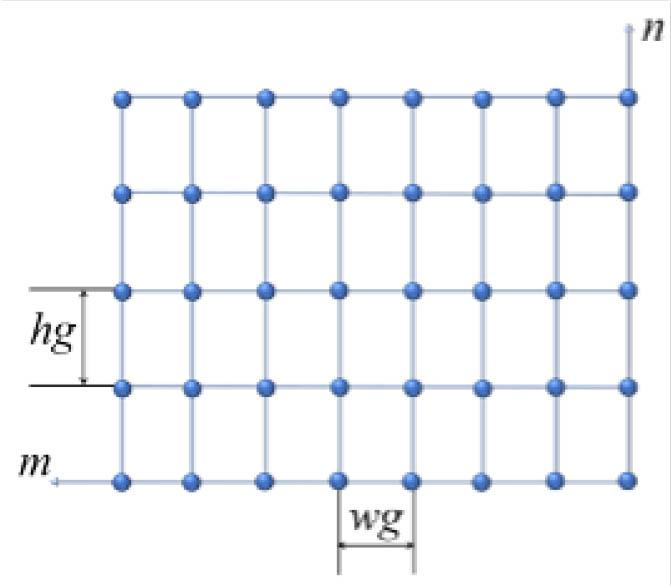Simplified particle systems for the fabric
Mass-spring model for overlaps

Double-bar reflex-lapping warp-knitted fabrics are formed by interconnected loops whose loop arc of the current course is connected with the loop root of the next course. Therefore, the position of a loop is determined by two particles P(m, n) controlling the loop root and P(m, n+1) controlling the loop arc. Springs linking these two particles are referred to as massless structural springs in the longitudinal direction as shown in Figure 2. However, there is no structural spring in the transverse direction because neighboring wales are connected by underlaps rather than overlaps.

Mass-spring model for underlaps

Underlaps of double-bar reflex-lapping warp-knitted fabrics are used for the connection of overlaps between adjacent courses whose length depends on both loop height and the number of wales they across. Different lengths of underlaps are subjected to different forces, and the corresponding shear springs are shown in Figure 3. It is also revealed in Figure 3 that one particle with a loop is acted upon by forces of two underlaps in one single bar.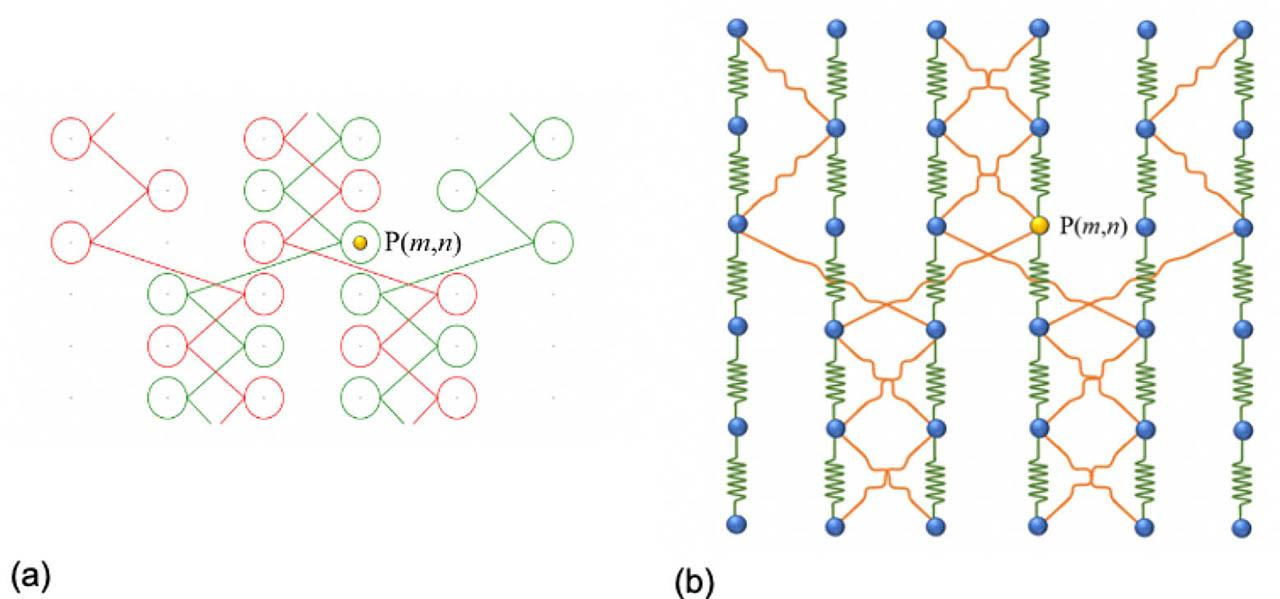A mass-spring model for double-bar reflex-lapping warp-knitted fabric with two repeats in weft direction: (a) lapping effect diagram, the red yarns, and the green yarns are threaded in two bars separately; (b) the mass-spring model correspondingly.
Structure deformation calculation

The warp-knitted fabric is simulated when laid on a flat platform so there is no consideration of outer forces, such as support forces, gravity, and so on. Only the internal forces between loops covering forces of structural springs, shear springs, and damping force are analyzed when studying factors for deformation. The forces can be calculated by the following equations [15,17]: $Fm,n=Fm,nst+Fm,nsh−Fm,nd$ {F_{m,n}} = F_{m,n}^{st} + F_{m,n}^{sh} - F_{m,n}^d $Fm,nst=−Pm,n+1−Pm,n|Pm,n+1−Pm,n|×(|Pm,n+1−Pm,n|−Hm,n−m,n+1)×kst$ F_{m,n}^{st} = - {{{P_{m,n + 1}} - {P_{m,n}}} \over {\left| {{P_{m,n + 1}} - {P_{m,n}}} \right|}} \times (\left| {{P_{m,n + 1}} - {P_{m,n}}} \right| - {H_{m,n - m,n + 1}}) \times {k_{st}} $Fm,nsh=−Pm+d,n+1−Pm,n|Pm+d,n+1−Pm,n|×(|Pm+diss,n+1−Pm,n|−lshear)×ksh$ F_{m,n}^{sh} = - {{{P_{m + d,n + 1}} - {P_{m,n}}} \over {\left| {{P_{m + d,n + 1}} - {P_{m,n}}} \right|}} \times (\left| {{P_{m + diss,n + 1}} - {P_{m,n}}} \right| - {l_{shear}}) \times {k_{sh}} $Fm,nd=−Pm,n(t)−Pm,n(t−Δt)Δt×kd$ F_{m,n}^d = - {{{P_{m,n}}(t) - {P_{m,n}}(t - \Delta t)} \over {\Delta t}} \times {k_d}

Where $Fm,nst$ F_{m,n}^{st} is the structural spring force between Pm,n and Pm,n+1, Hm,nm,n+1 is the length of this structural spring under forces, and kst is the structural spring coefficient. $Fm,nsh$ F_{m,n}^{sh} is the force of shear spring of Pm,n, Pm+d,n+1 · Pm+d,n+1 is the particle connected by the underlap starting from the loop on the particle Pm,n, and dis is the number of wales crossed by this particular underlap. lshear is the shear spring length under force, and ksh is the coefficient of the shear spring. $Fm,nd$ F_{m,n}^d is the damping force of the particle, Pm,n · Pm,n (t) is the position at current time t, and Pm,n (t − Δt) is the position at the last moment (t − Δt). t is the time step, and kd is damping coefficient. Comprehensively, Fm, n is the sum of all internal forces the particle Pm, n received.

The Verlet integration method is used to determine the particle position without calculation of particle velocity of the particle28. As shown in equation (7), the position of the particle at the future time (t − Δt) can be derived from the position at the last and the current moment, which leads to a more stable system. In equation (7), Mm,n is the particle mass. $Pm,n(t+Δt)=2×Pm,n(t)−Pm,n(t−Δt)+Fm,nMm,nΔt2$ {P_{m,n}}(t + \Delta t) = 2 \times {P_{m,n}}(t) - {P_{m,n}}(t - \Delta t) + {{{F_{m,n}}} \over {{M_{m,n}}}}\Delta {t^2}

During the movement, the kinetic energy of particles will go down continually because of the damping force and displacement will also decrease with it. The displacement threshold of particles is set as Lth in order to avoid overstretching of the spring and ensure the rationality of the deformation. Because excessive stretching of springs leads to yarn breakage, and excessive shift causes interpenetration between particles. If the particle displacement LLth, it means that particles are in a stable state and the deformation is completed.

Geometric loop model
Bezier curve model for loops

The Bezier curve has a powerful function for drawing smooth curves by any given point, and the loop path can be limited within the appropriate ranges to generate a more reasonable loop shape. In this paper, the quadratic Bezier curve with three control points shown in Figure 4 is selected to calculate the coordinate due to less amount of calculation. The equation of the quadratic Bezier curve is shown as equation (8). Q(t) represents the dynamic point at the moment t on the curve, and Q1-Q3 are the control points of the quadratic Bezier curve.

$Q(t)=(1−t)2Q1+2t(1−t)Q2+t2Q3$ Q(t) = {(1 - t)^2}{Q_1} + 2t(1 - t){Q_2} + {t^2}{Q_3}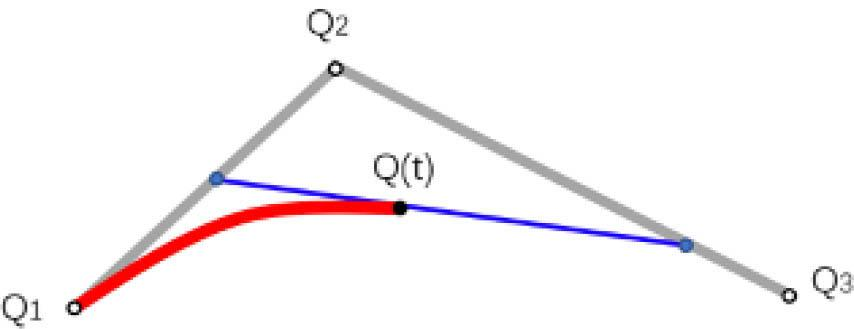The quadratic Bezier curve

Several control points are required to determine the basic shape of loops in the warp-knitted fabric in this paper as shown in Figure 5. The green dots (A0-A7) represent control points, while the blue ones (M1-M7) are the midpoints of each segment connected by two adjacent control points. A1-A2 andA5-A6 control two-loop limbs, and A2-A3-A4-A5 are for the loop arc. A0-A1 and A6-A7 are transition articulation curves linking the loops in adjacent courses. This figure also reveals that h is the height of the loop limb, which is the relaxed vertical distance of the particles in the warp direction. w is the width of the loop, b is the height of the loop arc, and d is the thick of the loop model. The loop model viewed from the front and the side are shown in Figure 5 (b) and (c) separately. Polylines connecting green dots are auxiliary lines to illustrate the position and size relationships between each controlling point. The frame of the actual stitch curves can be derived from points on these polylines. The overlap in warp-knitted fabric includes the loop limb and loop arc, which can be denoted as a smooth path composed of multiple Bezier curves as shown in Figure 6. The Bezier curves are generated automatically by the built-in geometry engine in the Three.js once the midpoints (M1-M7) are calculated.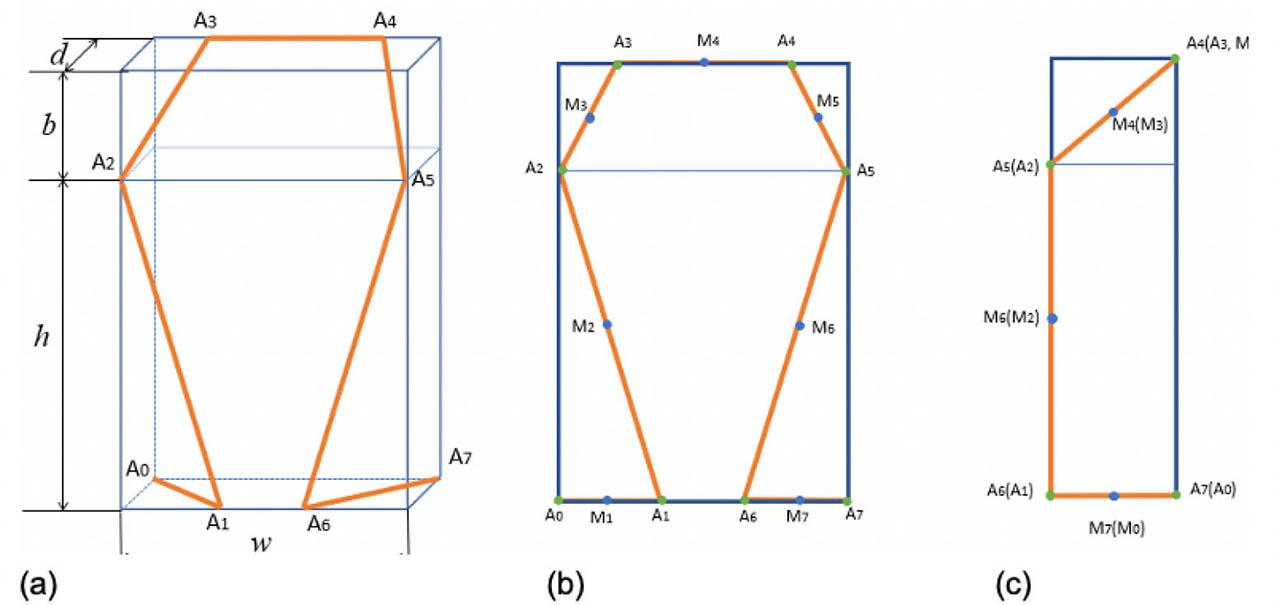A 3D model unit of a Warp-knitted loop: (a) 3D model of a loop; (b) Front view of the loop; (c) Side view of the loop.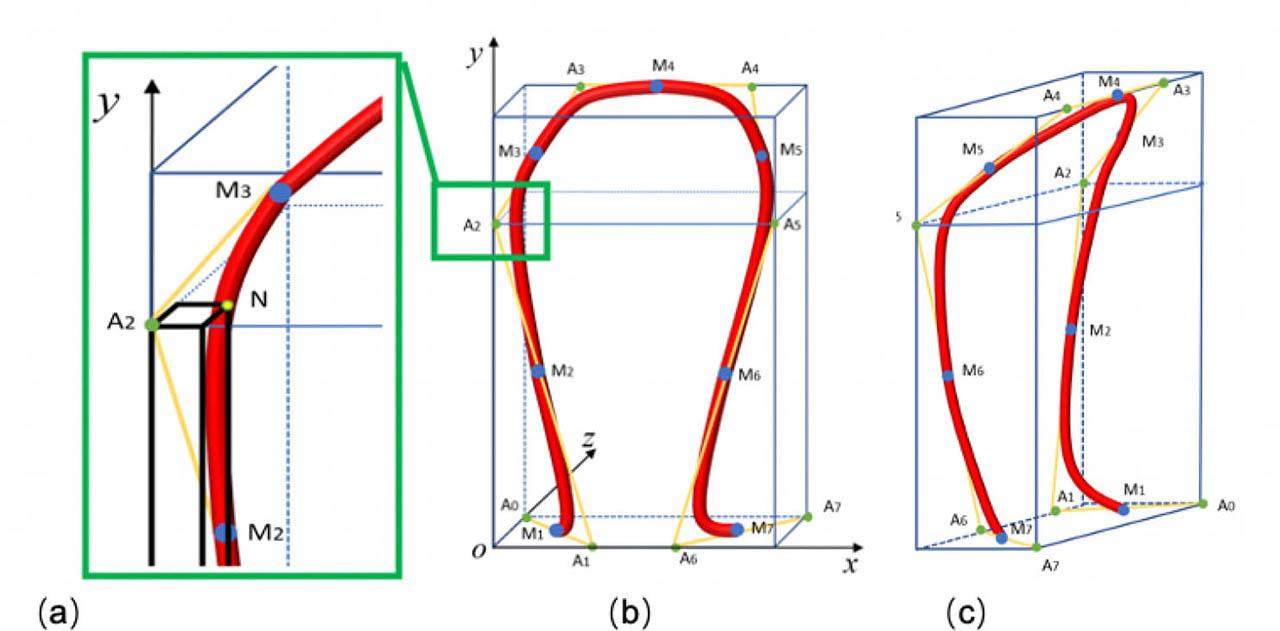3D model of a warp-knitted loop: (a) local minutiae of the loop curve, where N is the point on the Bezier curve, and M2-A2-M3 are control points of the second segment ; (b) the front view; (c): the side view.

The 3D loop model proposed in this paper consists of eight segments. Figure 7 is a plane graph of loops in two courses. Ai and Bi are the control points, and Mi is the midpoint of each segment. The underlaps are controlled by the last two points of the current loop (A6, A7) and the start two points of the loop in the next course (B0, B1). M1-A1-M2 is the first segment, and M1-A2-M3 is the second segment. And so on, for each of the seven segments, a complete loop model is composed.

Take the second segment M2-A2-M3 for example shown in Figure 6 (b). Assuming that the coordinate of M2 is (x1, y1, z1), A2 is (x2, y2, z2), M3 is (x3, y3, z3), and N on the curve is (xn, yn, zn). In particular, y coordinate of point N is the same as that of point A2. Thus, M2-A2-M3 is a Bezier curve through N so that the coordinate of N can be used in the quadratic Bezier curve equation. Calculation equations are as follows: $yn=y2$ {y_n} = {y_2} $M2=A1+A22$ {M_2} = {{{A_1} + {A_2}} \over 2} $M3=A2+A32$ {M_3} = {{{A_2} + {A_3}} \over 2}

Solving tn from equation (8) gives that $tn=(2y1−2y2)+4(y1−y2)2−4(y1−2y2+y3)×(y1−yn)2(y1−2y2+y3)$ {t_n} = {{(2{y_1} - 2{y_2}) + \sqrt {4{{({y_1} - {y_2})}^2} - 4({y_1} - 2{y_2} + {y_3}) \times ({y_1} - {y_n})} } \over {2({y_1} - 2{y_2} + {y_3})}}

Substituting the value of tn into the quadratic Bezier equation, values of xn and zn can be obtained as: $xn=(1−tn)2x1+2tn(1−tn)x2+tn2x3$ {x_n} = {(1 - {t_n})^2}{x_1} + 2{t_n}(1 - {t_n}){x_2} + {t_n}^2{x_3} $zn=(1−tn)2z1+2tn(1−tn)z2+tn2z3$ {z_n} = {(1 - {t_n})^2}{z_1} + 2{t_n}(1 - {t_n}){z_2} + {t_n}^2{z_3}

Other curves are calculated in a similar way. And space relationship of loops without shape deformation is shown in Figure 8. It is a common fabric knitted by two guide bars. The red yarns are threaded in the needle on the front bar (GB1), whose overlaps are on the technical face, and the underlaps are on the technical back. The green stitches are knitted by GB2, which are wrapped in the middle of the fabric.

Loop deformation with control points

The loop model established above is ideal in a relaxed state. When subjected to a force, the loop shape will deform to a certain extent. It is assumed that the force only acts in the x and y directions without changing the z-axis coordinate. As shown in Figure 9(a), the basic position of a loop is determined by particles P0 (m,n) and P1(m,n+1), which represent one particle on the wale m and the course n and the other particle on the wale m and course n+1. The warp-knitted fabric has a structure with loop interlocking, and the loop arc of the current course is connected to the root of the next course. Therefore, the position of the loop root is controlled by particle P0 (m,n), while the loop arc depends on P1(m,n+1). Figure 9(b) shows that the control points surrounding particles will move with the change of particles’ coordinates under forces. It is necessary to establish the relationship between the particles and the control points in order to better describe the shape change of the loop.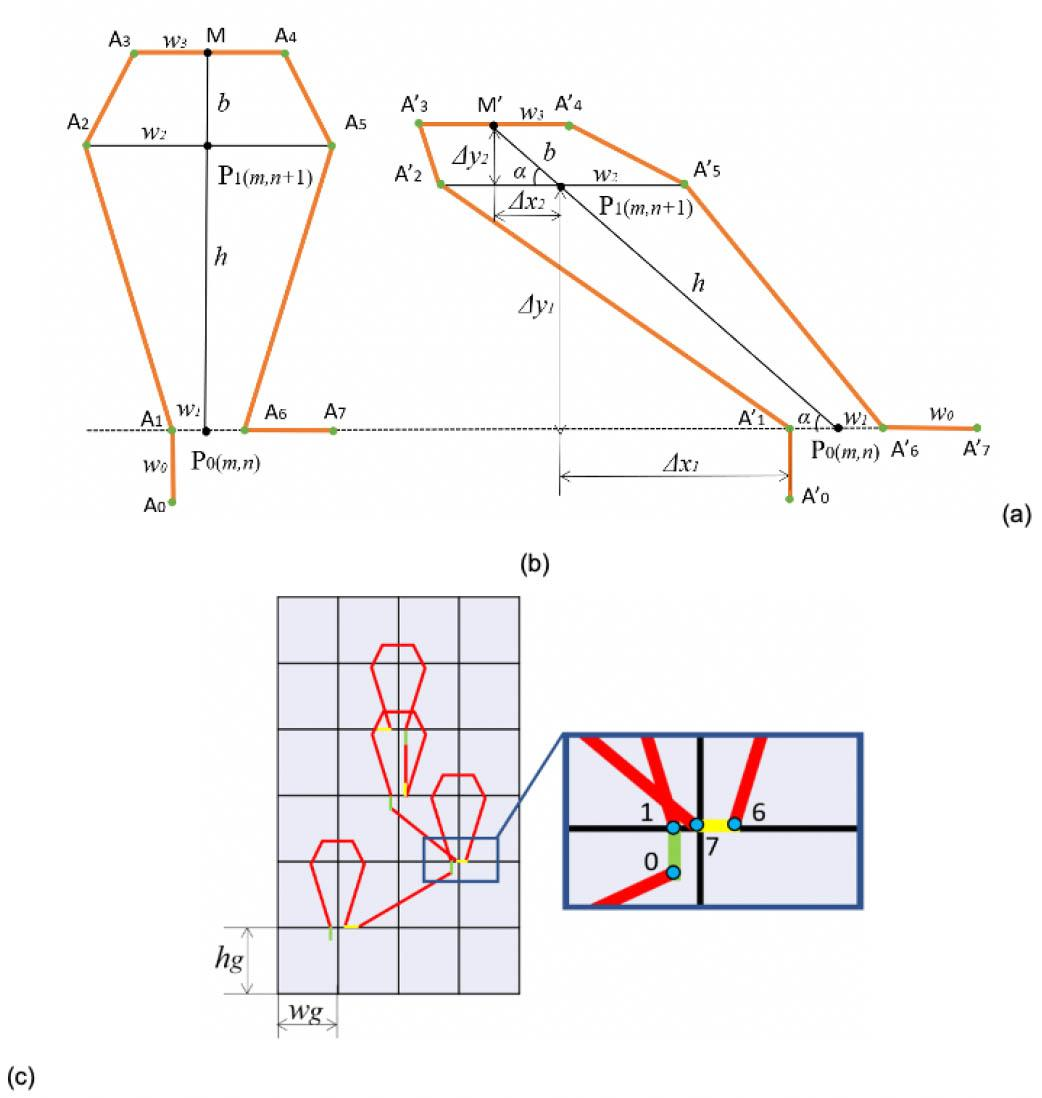Shape change based on the loop level: (a) the plain loop model in a relaxed state; (b) the plain loop model under forces; (c) plain loop models for different types of loops.

In Figure 9(a) and (b), A0-A7 are control points in the relaxed state, while A0’-A7’ are in one of the states under force. w1-w4 are relaxed distances between control points and the centerline. h and b are the relaxed height of the loop limb and the loop arc. When the loop is deformed, an inclination angle α is generated, which can be calculated by the coordinates of P0 and P1 as in equations (15)-(16). The length of P0-P1 and P1M’ remain unchanged as h and b. Δy1 and Δy2 are the vertical height of the loop limb and loop arc after deformation. Δx1 is the relative displacements to P1 horizontally, while Δx2 is that to P0. The values can be obtained by equations (17)(20). $tan α=Δy1Δx1=P1(y)−P0(y)P1(x)−P0(x)$ \tan \,\alpha = {{\Delta {y_1}} \over {\Delta {x_1}}} = {{{P_1}(y) - {P_0}(y)} \over {{P_1}(x) - {P_0}(x)}} $α=tan−1[P1(y)−P0(y)P1(x)−P0(x)]$ \alpha = {\tan ^{ - 1}}\left[ {{{{P_1}(y) - {P_0}(y)} \over {{P_1}(x) - {P_0}(x)}}} \right] $Δx1=P1(x)−P0(x)$ \Delta {x_1} = {P_1}(x) - {P_0}(x) $Δy1=P1(y)−P0(y)$ \Delta {y_1} = {P_1}(y) - {P_0}(y) $Δx2=bcosα$ \Delta {x_2} = b\cos \alpha $Δy2=bsinα$ \Delta {y_2} = b\sin \alpha

Values of the z axis of control points remain stable since only the position change in the X-Y plane is considered in this paper. But the need to pay attention to it is that the start point A0 and the endpoint A7 change dynamically. Referring to Figure 9 (c) for specific definitions, the green segments are the start of the loop, and the yellow ones are the segments linking to the next loop. The direction of them is affected by the loop types and position relationship of loops connected to it. The coordinates of control points are shown in Table 1.

Proportional relationship between control points and the loop structure parameters

Point x y z
M’ P1(x)+b *cosα P1(y)+b *sinα A3(z)
*A0 P0(x) - w1 P0(y)-w0 A0(z)
A1 P0(x) - w1 P0(y) A1(z)
A2 P1(x) - w2 P1(y) A2(z)
A3 P1(x)+b *cosα-w3 P1(y)+b *sinα A3(z)
A4 P1(x)+b *cosα+w3 P1(y)+b *sinα A4(z)
A5 P1(x)+w2 P1(y) A5(z)
A6 P0(x)+w1 P0(y) A6(z)
*A7 P0(x)+(w0 +w1) P0(y) A7(z)

Note that A0 and A7 with ‘*’ mean that they change dynamically.

Results and discussion

The simulator proposed in this paper is designed by Visual Studio 2015. Using C#, a kind of computer language, the mass-spring model and warp-knitted loop models are established, and key parameters, such as the force, displacement of particles, and coordinate of loop control points, are calculated. Due to the control points of loops computed by two vertical neighboring particles, each loop can be deformed along with the displacement of these related particles. Once getting the point coordinates, smooth tubes are generated along a specific path with three.js technology. Ambient light and directional light are used to cause shadings so that the stereo feeling of yarns can be reflected, realizing the simulation of double-bar reflex-lapping warp-knitted fabrics with chemical filaments. The flow chart of deformation simulation is as Figure 10 shows.

In order to compare the influence of different chain notations on fabric structure deformation, two pieces of double-bar reflex-lapping fabrics using the same yarns are selected. The technical parameters are shown in Table 2. The particle displacement results are shown in Figure 11. Due to the lateral displacement of the warp-knitting bars, some laps of the pattern move more than one wales. Particularly on the leftmost yarn moves beyond the particle model, loops out of range need to be constrained on particles at the edge to ensure the model in regular.

Basic parameters of two fabrics

No. Chain notation Yarn specification Threading
1 GB1:1-0/1-2//;GB2:1-2/1-0//; A: nylon in 22dtex/1F, pinkB: nylon in 22dtex/1F, blue GB1:1A; GB2:1B.
2 GB1:1A, 1*; GB2:2B.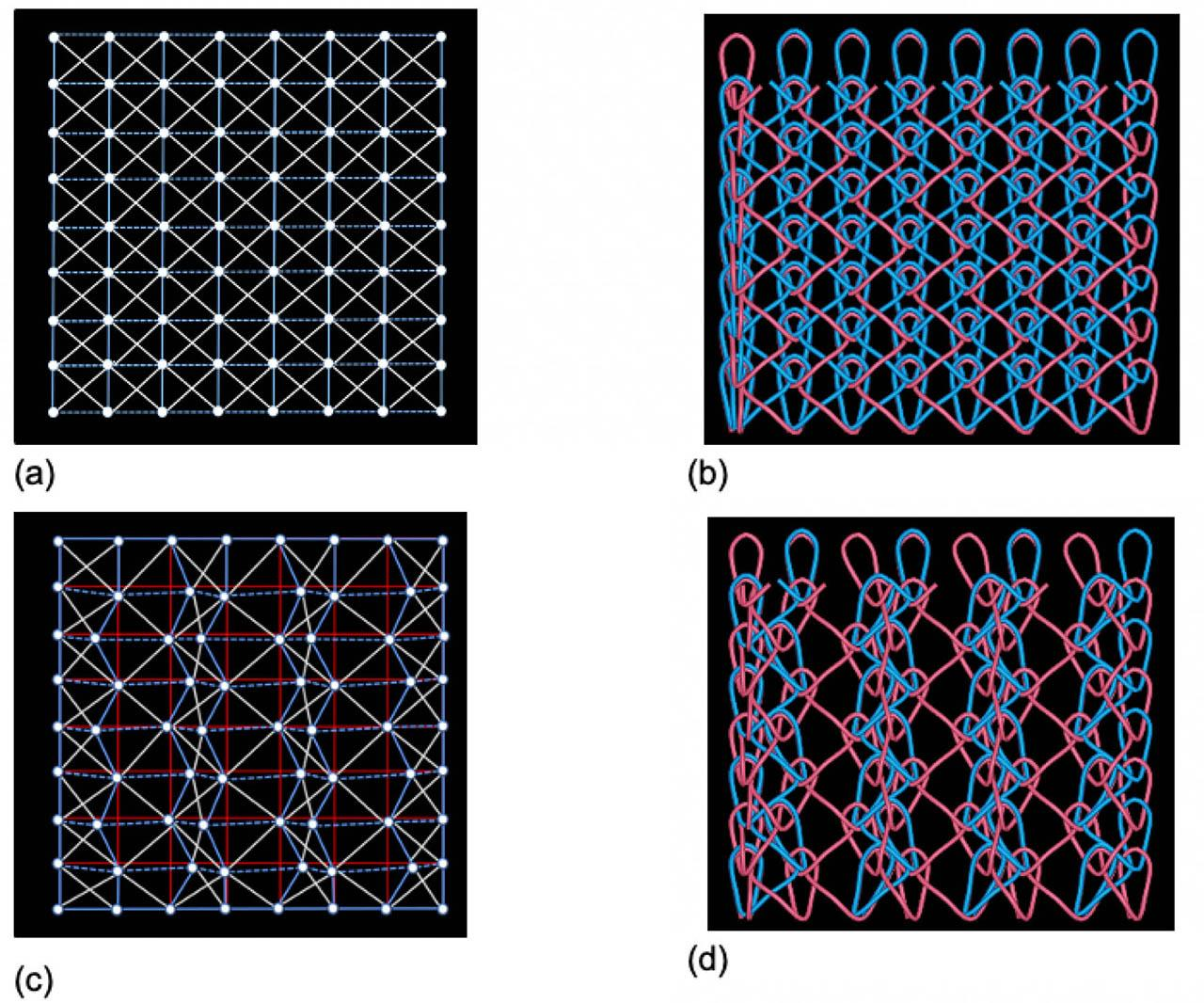Deformation comparison of fabrics knitted by double-bar machines: (a), (b) represent the simulation of fabric 1 and (c), (d) represent the simulation of fabric 2.

When the particles are in equilibrium as shown in Figure 11 (a) and (b), particles remain at the original position without offset, and loops are in an ideal upright state. However, Figure 11 (c) and (d) illustrate if there are some needles without yarn threading, which means some particles are in the forces of two loops and some are in the forces of only one loop, there will be an imbalance in the force on particles, and displacement will cause the deformation of the fabric. In Figure 11 (c), the red mesh represents the initial position without deformation. Based on this, the irregular blue mesh with white particles shows the deviation results.

Another example is taken to illustrate the effect of the parameter change on fabric deformation and the real-time simulation. A pattern repeat contains six courses and two wales knitted on a high-speed tricot warp-knitting machine HKS2 (E28) produced by KARL MAYER (Germany). The basic parameters are listed in Table 3.

Parameters of fabric knitted by HKS2 (E28)

Pattern width 2 wales Pattern height 4 courses
Finished horizontal density 9 wpc Finished longitudinal density 11.2 cpc
Run-in values GB1:1800mm/rack; GB2:1800mm/rack;
Chain notation GB1:2-3/2-1/2-3/1-0/1-2/1-0//;GB2:1-0/1-2/1-0/2-3/2-1/2-3//;
Materials A:76dtex/24F, polyester;

After the technical parameters are set, the simulation is conducted according to the flow chart. Four figures show the fabrics in the view dimensions of 234.96 mm and 83.75 mm. The real sample and the simulation result of the one-one ratio are shown in Figure 12 (a) and (b). The fabric contains about 13 diamonds in the course direction and about 6.5 diamonds in the wale direction. To test the real-time simulation, one of the technical parameters is changed to resimulation and compared with real samples. Figure 12 (c) shows the simulation with finished horizontal density in 6 wpc and finished longitudinal density in 11.2 cpc, which contains about 8.3 diamonds in the course direction and about 6.5 diamonds in the wale direction. While Figure 12 (d) shows the finished density in 9 wpc and 11.2 cpc with about 20 diamonds in the course direction and about 6.5 diamonds in the wale direction. The final simulated deformation effect is displayed once the parameter is changed.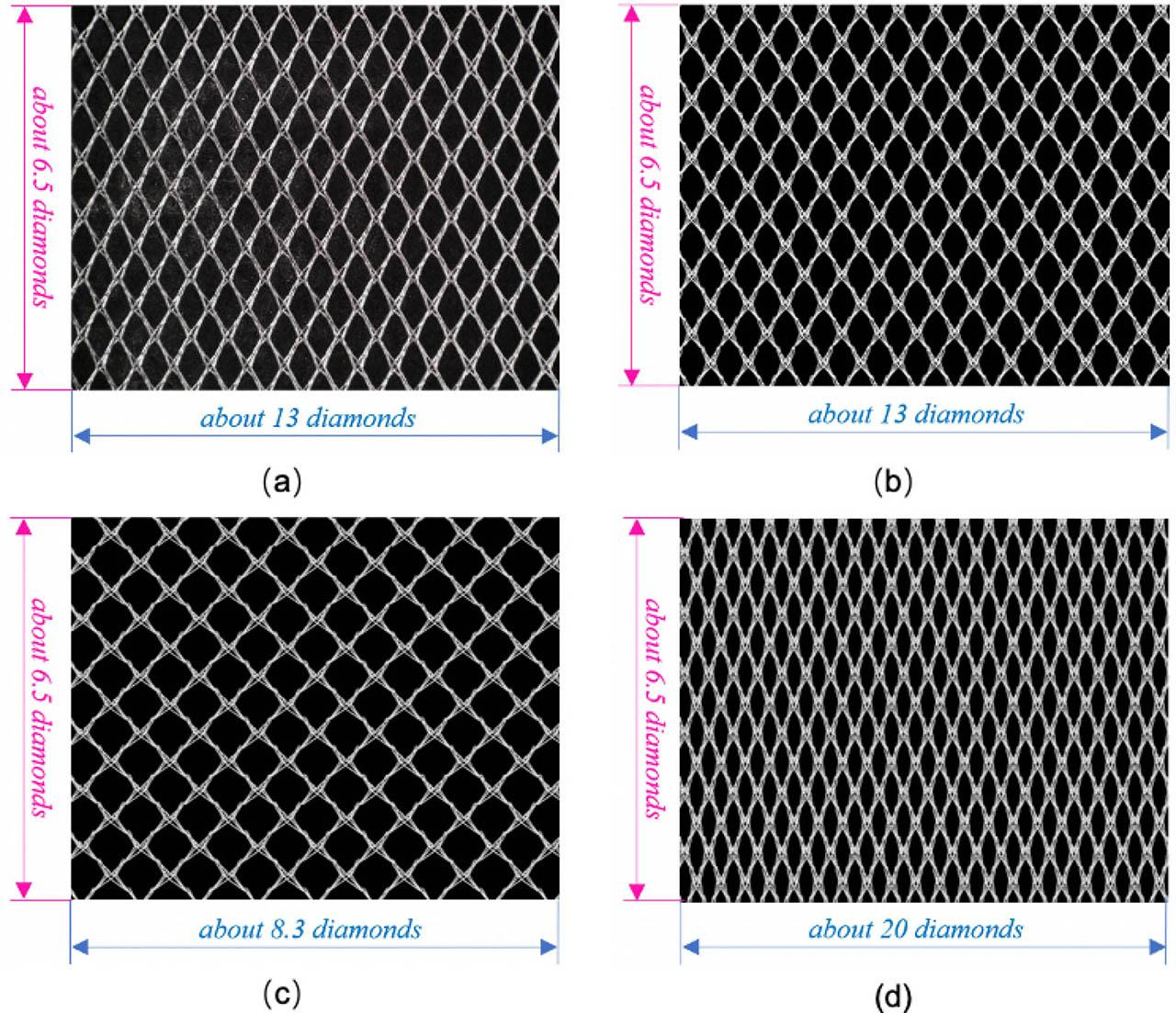The real sample and the final results with different parameters: (a) a real sample; (b) a simulation in real parameters; (c) a simulation in 6 wpc and 11.2 cpc; (d) a simulation in 9 wpc and 7 cpc.

Figure 13 are the simulation effects of the same part with actual knitting parameters. The data in Table 4 are the average value of 50 measurements for different parts of the real sample, and the measurement values of simulation are generated automatically. The specific measuring position is marked in Figure 13. P1-P4 are four vertices of the diamond, while <α and <β are two adjacent inside vertex angles of the diamond. As revealed from Table 4, the deviations between the simulation and the real sample are small, which indicate the deformation simulator has high accuracy.

Comparison between simulation and the real sample

Tested pairs Simulation Sample Deviation
P1-P3 12.5 mm 12.9 mm 3.10%
P2-P4 17.8 mm 18.1 mm 1.58%
<α 118.127° 118.902° 0.65%
<β 64.514° 64.973° 0.70%
Conclusion

A deformation simulation method with a simplified mass-spring model for double-bar reflex-lapping warp-knitted fabrics is proposed in this paper. Structural springs and shear springs are, respectively, denoted as yarns of loop limbs and underlaps based on the warp-knitted technical characteristics. The forces on particles are analyzed, and the particles’ motion state and deformations are calculated based on Verlet integral. Besides, a simple model for hyperelastic detection of particles is established in order to improve the accuracy and rationality of particle movement. The loop path is generated by quadratic Bezier curves, and dynamic models for loops and inlays are built. The control points of each loop in a deformation state are calculated according to the relationship of the particles and control points. The validity and correctness of the spring particle model to simulate the deformation behavior are verified by calculating the deformation ratio analysis. C# and JavaScript via web technology were used to implement the deformation simulation and display of warp-knitted fabrics. Furthermore, the simulation results change with different parameters, which is helpful for the designer to modify the parameters before knitting. It also has obviously exhibited time and cost savings and greater practicability.

#### Comparison between simulation and the real sample

Tested pairs Simulation Sample Deviation
P1-P3 12.5 mm 12.9 mm 3.10%
P2-P4 17.8 mm 18.1 mm 1.58%
<α 118.127° 118.902° 0.65%
<β 64.514° 64.973° 0.70%

#### Parameters of fabric knitted by HKS2 (E28)

Pattern width 2 wales Pattern height 4 courses
Finished horizontal density 9 wpc Finished longitudinal density 11.2 cpc
Run-in values GB1:1800mm/rack; GB2:1800mm/rack;
Chain notation GB1:2-3/2-1/2-3/1-0/1-2/1-0//;GB2:1-0/1-2/1-0/2-3/2-1/2-3//;
Materials A:76dtex/24F, polyester;

#### Proportional relationship between control points and the loop structure parameters

Point x y z
M’ P1(x)+b *cosα P1(y)+b *sinα A3(z)
*A0 P0(x) - w1 P0(y)-w0 A0(z)
A1 P0(x) - w1 P0(y) A1(z)
A2 P1(x) - w2 P1(y) A2(z)
A3 P1(x)+b *cosα-w3 P1(y)+b *sinα A3(z)
A4 P1(x)+b *cosα+w3 P1(y)+b *sinα A4(z)
A5 P1(x)+w2 P1(y) A5(z)
A6 P0(x)+w1 P0(y) A6(z)
*A7 P0(x)+(w0 +w1) P0(y) A7(z)

#### Basic parameters of two fabrics

No. Chain notation Yarn specification Threading
1 GB1:1-0/1-2//;GB2:1-2/1-0//; A: nylon in 22dtex/1F, pinkB: nylon in 22dtex/1F, blue GB1:1A; GB2:1B.
2 GB1:1A, 1*; GB2:2B.

Peirce, F. T. (1947). Geometrical principles applicable to the design of functional fabrics. Textile Research Journal, 17(3), 123–147. PeirceF. T. 1947 Geometrical principles applicable to the design of functional fabrics Textile Research Journal 17 3 123 147 10.1177/004051754701700301 Search in Google Scholar

Provot, X. (1995). Deformation constraints in a Mass-Spring Model to describe rigid cloth behavior. Proceedings of the 1995 Graphic Interface Conference, 147–155. ProvotX. 1995 Deformation constraints in a Mass-Spring Model to describe rigid cloth behavior Proceedings of the 1995 Graphic Interface Conference 147 155 Search in Google Scholar

Provot, X. (1997). Collision and self-collision handing in cloth model dedicated to design garment. Proceedings of the 1997 Graphics Interface Conference, 177–189. ProvotX. 1997 Collision and self-collision handing in cloth model dedicated to design garment Proceedings of the 1997 Graphics Interface Conference 177 189 Search in Google Scholar

Hui, W. H., Guan Z. X. (2007). Research on cloth modeling simulation based on mass spring model. Computer Engineering and Design, (3), 664–667. HuiW. H. GuanZ. X. 2007 Research on cloth modeling simulation based on mass spring model Computer Engineering and Design 3 664 667 Search in Google Scholar

Wang, J. C., Hu, X.R., Wang H. (2008). Simulation technology of cloth modeling based on mass spring model. Journal of Wuhan University of Science and Engineering, (9), 5–8. WangJ. C. HuX.R. WangH. 2008 Simulation technology of cloth modeling based on mass spring model Journal of Wuhan University of Science and Engineering 9 5 8 Search in Google Scholar

Hu, J. F., Yan, P. M., Zhou, J. W., et al. (2008). Real-time cloth simulation based on improved mass-spring model. Computer Simulation, (7), 184–187. HuJ. F. YanP. M. ZhouJ. W. 2008 Real-time cloth simulation based on improved mass-spring model Computer Simulation 7 184 187 Search in Google Scholar

Ji, F., Li, R. Q., Qiu, Y. P. (2007). Simulate the Dynamic Draping Behavior of Woven and Knitted Fabrics. Journal of Industrial Textiles, 35(3), 201–215. JiF. LiR. Q. QiuY. P. 2007 Simulate the Dynamic Draping Behavior of Woven and Knitted Fabrics Journal of Industrial Textiles 35 3 201 215 10.1177/1528083706055753 Search in Google Scholar

Choi, K. J., Ko, H. S. (2002). Stable but responsive cloth. ACM Transactions on Graphics, 21(3), 604–611. ChoiK. J. KoH. S. 2002 Stable but responsive cloth ACM Transactions on Graphics 21 3 604 611 10.1145/566654.566624 Search in Google Scholar

Liu, L. X., Song, Q. (2011). Cloth-object deformation simulation based on a simplified mass-spring model. Computer Simulation, (5), 406–409. LiuL. X. SongQ. 2011 Cloth-object deformation simulation based on a simplified mass-spring model Computer Simulation 5 406 409 Search in Google Scholar

Mozafary, V., Payvandy, P. (2017). A novel method based on loop shape for simulating knitted fabric using mass spring model. Fibers and Polymers, 18(3), 533–541. MozafaryV. PayvandyP. 2017 A novel method based on loop shape for simulating knitted fabric using mass spring model Fibers and Polymers 18 3 533 541 10.1007/s12221-017-1066-0 Search in Google Scholar

Mozafary, V., Payvandy, P., Rezaeian, M. (2018). A novel approach for simulation of curling behavior of knitted fabric based on mass spring model. Journal of the Textile Institute, 109(12), 1620–1641. MozafaryV. PayvandyP. RezaeianM. 2018 A novel approach for simulation of curling behavior of knitted fabric based on mass spring model Journal of the Textile Institute 109 12 1620 1641 10.1080/00405000.2018.1453635 Search in Google Scholar

Güdükbay, U., Bayraktar, S., Koca, Ç., et al. (2014). Particle-based simulation of the interaction between fluid and knitwear. Signal Image and Video Processing, 8(3), 415–422. GüdükbayU. BayraktarS. KocaÇ. 2014 Particle-based simulation of the interaction between fluid and knitwear Signal Image and Video Processing 8 3 415 422 10.1007/s11760-012-0308-2 Search in Google Scholar

Dabiryan, H., Ashouri, M. H. (2020). Mesoscale model for elastic modulus of warp-knitted fabric reinforced composites based on spring model. Journal of Industrial Textiles, 50(1), 46–69. DabiryanH. AshouriM. H. 2020 Mesoscale model for elastic modulus of warp-knitted fabric reinforced composites based on spring model Journal of Industrial Textiles 50 1 46 69 10.1177/1528083718821873 Search in Google Scholar

Sha, S., Jiang, G. M., Ma, P. B., (2015). et al. 3-D dynamic behaviors simulation of weft knitted fabric based on particle system. Fibers and Polymers, 16(8), 1812–1817. ShaS. JiangG. M. MaP. B. 2015 3-D dynamic behaviors simulation of weft knitted fabric based on particle system Fibers and Polymers 16 8 1812 1817 10.1007/s12221-015-5254-5 Search in Google Scholar

Sha, S., Ma, P. B., Chapman, L. P., et al. (2017). Three-dimensional modeling and simulation of deformation behavior of fancy weft knitted stitch fabric. Textile Research Journal, 87(14), 1742–1751. ShaS. MaP. B. ChapmanL. P. 2017 Three-dimensional modeling and simulation of deformation behavior of fancy weft knitted stitch fabric Textile Research Journal 87 14 1742 1751 10.1177/0040517516658518 Search in Google Scholar

Sha, S., Jiang, G. M., Chapman, L. P., et al. (2017). Fast penetration resolving for weft knitted fabric based on collision detection. Journal of Engineered Fibers and Fabrics, 12(1), 50–58. ShaS. JiangG. M. ChapmanL. P. 2017 Fast penetration resolving for weft knitted fabric based on collision detection Journal of Engineered Fibers and Fabrics 12 1 50 58 10.1177/155892501701200106 Search in Google Scholar

Peng, J. J., Jiang, G. M., Xia, F. L., et al. (2020). Deformation and geometric modeling in three-dimensional simulation of fancy weft-knitted fabric. Textile Research Journal, 90(13–14), 1527–1536. PengJ. J. JiangG. M. XiaF. L. 2020 Deformation and geometric modeling in three-dimensional simulation of fancy weft-knitted fabric Textile Research Journal 90 13–14 1527 1536 10.1177/0040517519894749 Search in Google Scholar

Arumugam, V., Mishra, R., Militky, J., et al. (2016). In-plane shear behavior of 3D spacer knitted fabrics. Journal of Industrial Textiles, 46(3), 868–886. ArumugamV. MishraR. MilitkyJ. 2016 In-plane shear behavior of 3D spacer knitted fabrics Journal of Industrial Textiles 46 3 868 886 10.1177/1528083715601509 Search in Google Scholar

Zhang, L. Z., Jiang, G. M. (2010). Loop offset of warp-knitted structures in 3-D simulation. Journal of Textile Research, 31(3), 64–67. ZhangL. Z. JiangG. M. 2010 Loop offset of warp-knitted structures in 3-D simulation Journal of Textile Research 31 3 64 67 Search in Google Scholar

Guo, C. X. (2018). The study of structure and deformation of warp-knitted mesh fabric with less guide bars. Wuhan Textile University, Wuhan, China. GuoC. X. 2018 The study of structure and deformation of warp-knitted mesh fabric with less guide bars Wuhan Textile University Wuhan, China Search in Google Scholar

Deng, W., Wang, T. T., Deng, Z. M. (2011). Mass-spring models used in jacquard fabric. Journal of Wuhan University of Science and Engineering, 24(3), 74–78. DengW. WangT. T. DengZ. M. 2011 Mass-spring models used in jacquard fabric Journal of Wuhan University of Science and Engineering 24 3 74 78 Search in Google Scholar

Li, X. X., Zhang, A. J., Ma, P. B., et al. (2017). Structural deformation behavior of Jacquardtronic lace based on the mass-spring model. Textile Research Journal, 87(10), 1242–1250. LiX. X. ZhangA. J. MaP. B. 2017 Structural deformation behavior of Jacquardtronic lace based on the mass-spring model Textile Research Journal 87 10 1242 1250 10.1177/0040517516651103 Search in Google Scholar

Chen, Y., Wu, M. Z., Hu, X. R. (2013). An improved mass-spring cloth model and simulation. Journal of South China Normal University(Natural Science Edition), 45(5), 43–46. ChenY. WuM. Z. HuX. R. 2013 An improved mass-spring cloth model and simulation Journal of South China Normal University(Natural Science Edition) 45 5 43 46 Search in Google Scholar

Mozatary, V., Payvandy, P. (2016). Study and comparison techniques in fabric simulation using mass spring model. International Journal of Clothing Science and Technology, 28(5), 634–689. MozataryV. PayvandyP. 2016 Study and comparison techniques in fabric simulation using mass spring model International Journal of Clothing Science and Technology 28 5 634 689 10.1108/IJCST-03-2015-0033 Search in Google Scholar

Baraff, D., Witkin, A. (1998). Large Steps in Cloth Simulation. Proceedings of Siggraph, Orlando, 43–54. BaraffD. WitkinA. 1998 Large Steps in Cloth Simulation Proceedings of Siggraph Orlando 43 54 10.1145/280814.280821 Search in Google Scholar

Ng, H. N., Grimsdale, R. L. (1995). Image Analysis Applications and Computer Graphics. Lecture Notes in Computer Science, Springer-verlag, Berlin, 1024, 124–331. NgH. N. GrimsdaleR. L. 1995 Image Analysis Applications and Computer Graphics Lecture Notes in Computer Science, Springer-verlag, Berlin 1024 124 331 10.1007/3-540-60697-1_95 Search in Google Scholar

Kubiak, B., Pietroni, N., Ganovelli, F., et al. (2007). A Robust Method for Real-time Thread Simulation. Proceedings of the ACM VRST, California, 85–88. KubiakB. PietroniN. GanovelliF. 2007 A Robust Method for Real-time Thread Simulation Proceedings of the ACM VRST California 85 88 10.1145/1315184.1315198 Search in Google Scholar

Mongus, D., Repnik, B., Mernik, M., et al. (2012). A hybrid evolutionary algorithm for tuning a cloth-simulation model. Applied Soft Computing, 12(8): 266–273. MongusD. RepnikB. MernikM. 2012 A hybrid evolutionary algorithm for tuning a cloth-simulation model Applied Soft Computing 12 8 266 273 10.1016/j.asoc.2011.08.047 Search in Google Scholar

• #### Archaeology and Virtual Simulation Restoration of Costumes in the Han Xizai Banquet Painting

Recommended articles from Trend MD